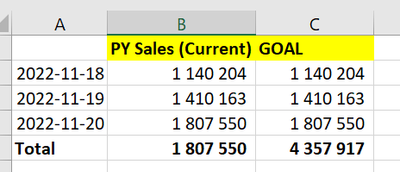cancel
Showing results for
Did you mean:Helper I

## Previous Year Calculation -1 day

Hi!

So I'm trying to "move" alla previous year metrics one day.  For example; 2022-11-18 should be compared to 2021-11-19. I have solved it on a line by line basis but the sum of days doesn't work. I realize that it must be down to my "max"-part of the calculation but can't figure out an alternative to work.

See below example, the total takes the latest date.Formula looks like this:

CALCULATE([Sales], FILTER(ALL('Date'[Date]), 'Date'[Date]=MAX(OrderData[OrderDate])-364))

The goal is for PY Sales to look like this:Any ideas?

1 ACCEPTED SOLUTIONSuper User

Ok, try this then:

``````PY =
SUMX (
VALUES ( Table[Date] ),
CALCULATE (
[Sales],
FILTER (
ALL ( 'Date'[Date] ),
'Date'[Date]
= MAX ( OrderData[OrderDate] ) - 364
)
)
)
``````

In doing so, you are also helping me. Thank you!

Proud to be a Super User!

4 REPLIES 4Super User

Try:

``````PY with total =
SUMX(VALUES(Table[Date]), [PY Sales])``````

In doing so, you are also helping me. Thank you!

Proud to be a Super User!Helper I

Hi! The solution works, but its' not ideal because then I need to create 2 metrics for all PY calculations.

In other words, ideally I would just have 1 metric for PY rather than having to create first the "YTD"* PY calculation and then the sum calculation of that.

*YTD = Max date being last orderdateSuper User

Ok, try this then:

``````PY =
SUMX (
VALUES ( Table[Date] ),
CALCULATE (
[Sales],
FILTER (
ALL ( 'Date'[Date] ),
'Date'[Date]
= MAX ( OrderData[OrderDate] ) - 364
)
)
)
``````

In doing so, you are also helping me. Thank you!

Proud to be a Super User!Helper I

@PaulDBrown Thank you!